Question

Assume that the transition matrix from basis B = {b1, b2, b3} to basis C = {c1, c2, c3} is PC,B = 1/2*[ 0 -1 1 ; -1 1 1 ; 1 0 0 ].

(a) If u = b1 + b2 + 2b3, find [u]C.

(b) Calculate PB,C.

(c) Suppose that c1 = (1, 2, 3), c2 = (1, 2, 0), c3 = (1, 0, 0) and let S be the standard basis for R 3 . (i) Find PS,B. (ii) Using part (i), determine the explicit form of the vectors b1, b2, b3.

2. (a). u = b1+b2+2b3 so that [u]B = (1,1,2)T.

[u]C = PC,B[u]B = PC,B( 1,1,2)T = (1/2,1,1/2).T

(b). PB,c = (PC,B)-1 =

 0 0 2 -1 1 1 1 1 1

( c). Since PC,B =

 0 -1/2 1/2 -1/2 1/2 1/2 1/2 0 0

Hence, b1 = 0.c1-(1/2)c2 +(1/2)c3 = -(1/2)(1,2,0) +(1/2)(1,0,0) = (0,-1,0), b2 = -(1/2).c1+(1/2)c2 +0c3 = -(1/2)(1,2,3)+(1/2)(1,2,0) = (0,0,-3/2) and , b3 = (1/2)c1+(1/2)c2 +0c3 = (1/2)(1,2,3)+(1/2)(1,2,0)= (1,2,3/2).

Let A =

 1 0 0 0 0 1 0 1 0 -1 0 2 0 0 1 0 -3/2 3/2

Hence PS, B =

 0 0 1 -1 0 2 0 -3/2 3/2

b1 =(0,-1,0), b2 = (0,0,-3/2) and , b3 =(1,2,3/2).

#### Earn Coins

Coins can be redeemed for fabulous gifts.

Similar Homework Help Questions
• ### 1) for R2 Given the vectors b1,b2, C1, and cz. B = {b1,b2} is a basis...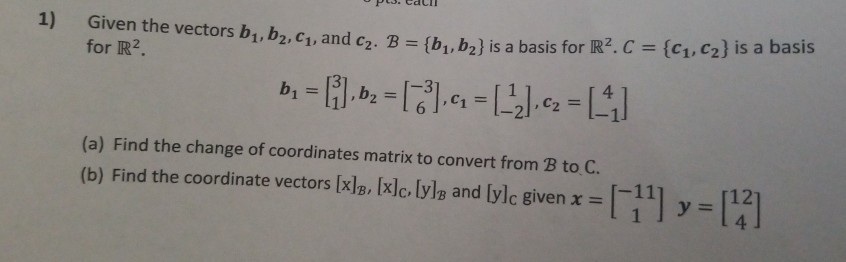1) for R2 Given the vectors b1,b2, C1, and cz. B = {b1,b2} is a basis for R2C = {C1,C2} is a basis b = [i.bz = ,4 = (-2) c2 =  (a) Find the change of coordinates matrix to convert from B to C. (b) Find the coordinate vectors [x]B, [x]c, lyle and [ylc given x =  y = 

• ### (b) Let E = {(1, C2, C3} be the standard basis for R3, B = {bų,...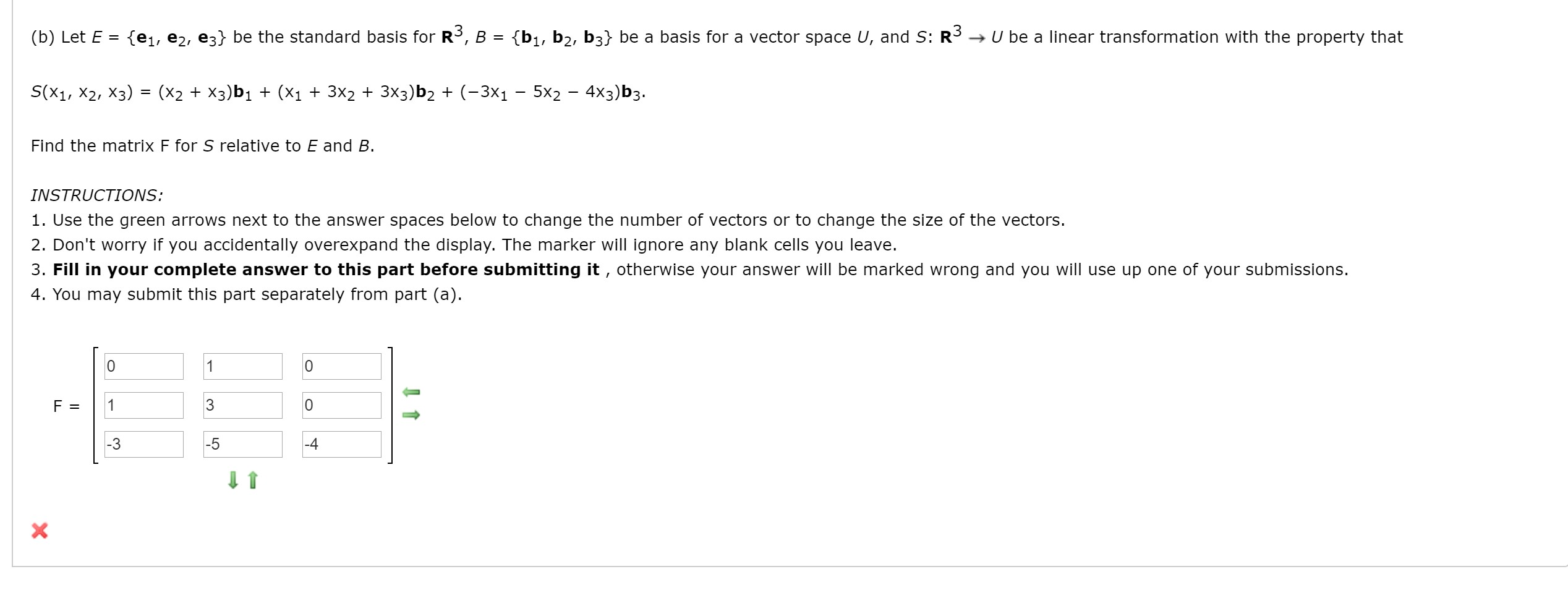(b) Let E = {(1, C2, C3} be the standard basis for R3, B = {bų, b2, b3} be a basis for a vector space U, and S: R3 → U be a linear transformation with the property that S(X1, X2, X3) (x2 + x3)b1 + (x1 + 3x2 + 3x3)b2 + (-3X1 - 5x2 - 4x3)b3. Find the matrix F for S relative to E and B. INSTRUCTIONS: 1. Use the green arrows next to the answer spaces below...

• ### Let B = {b1,b2, b3} be a basis for a vector space V. Let T be...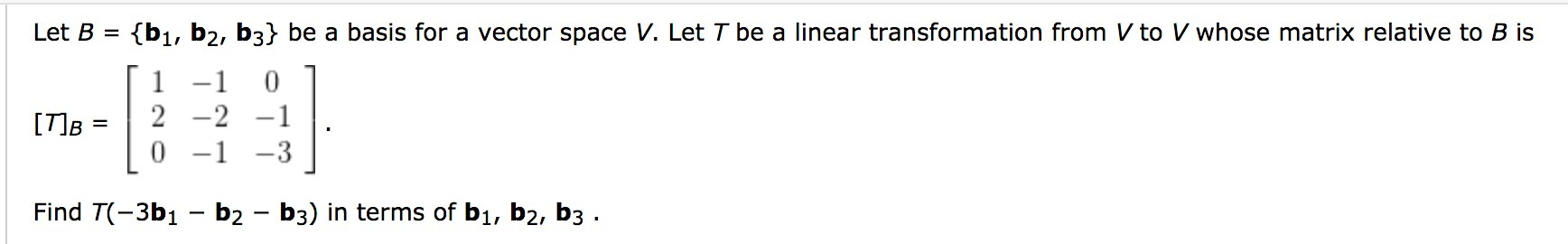Let B = {b1,b2, b3} be a basis for a vector space V. Let T be a linear transformation from V to V whose matrix relative to B is [ 1 -1 0 1 [T]B = 2 -2 -1 . 10 -1 -3 1 Find T(-3b1 – b2 - b3) in terms of bı, b2, b3 .

• ### 9. Let S = {C1, C2, C3, es} be the standard basis for R, and let...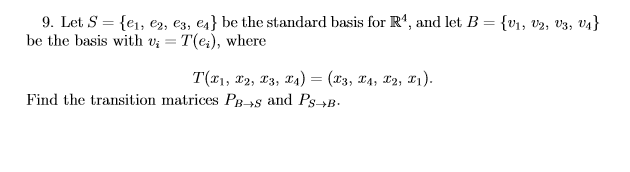9. Let S = {C1, C2, C3, es} be the standard basis for R, and let B = {V1, 02, 03, 04} be the basis with vi = T(e), where T(21, 12, 13, 14) = (x3, 14, 20, 21). Find the transition matrices PB +and Ps+B.

• ### 0 1 Let S span 1 1 1 0 }, a basis for S. Show that| (a) Let B1 { 1 0 1 1 0 is also a basis for S 0 B2 { 1 (b) Write eac...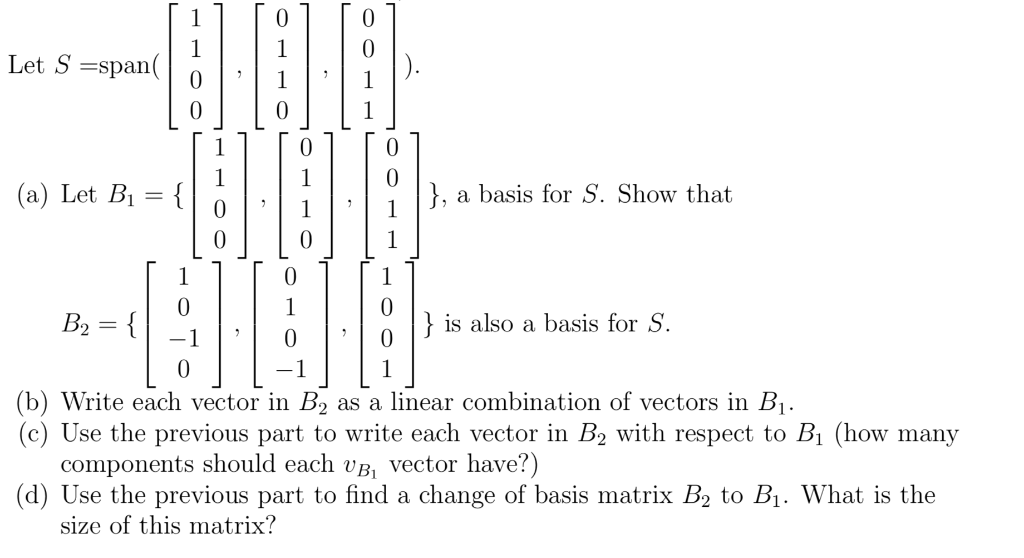0 1 Let S span 1 1 1 0 }, a basis for S. Show that| (a) Let B1 { 1 0 1 1 0 is also a basis for S 0 B2 { 1 (b) Write each vector in B2 (c) Use the previous part to write each vector in B2 with respect to Bi (how many components should each vB, vector have?) (d) Use the previous part to find a change of basis matrix B2 to B1. What...

• ### solution of question d (4 points) Consider the basis of R5 given by with b2 (2,-1,-5,-4,7), b3-(3, 2,-7,-5,9) b4 2,1,4,...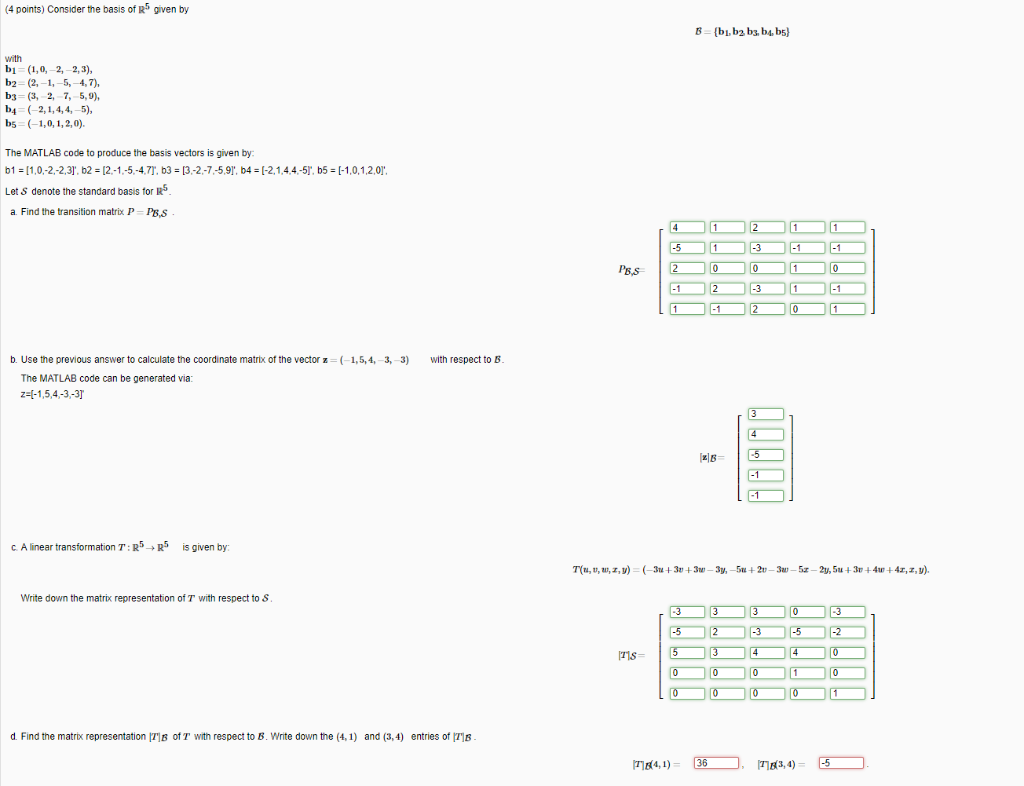solution of question d (4 points) Consider the basis of R5 given by with b2 (2,-1,-5,-4,7), b3-(3, 2,-7,-5,9) b4 2,1,4,4,-5) bs (-1,0,1,2,0) The MATLAB code to produce the basis vectors is given by b1 11,0-2-2.3], b2 -12-1.-5-4,7T, b3 13-2-7-5,91, b4 [-2,14.4-5T, b5 1-1,0,1,20 Let S denote the standard basis for R a Find the transition matrix P P,s PB,s b. Use the previous answer to calculate the coordinate matrix of the vector z ( 1,5, 4, 3, 3) with respect...

• ### Problem 4. Determine if the following sets B1, B2, B3, B4 and Bs are open, closed,...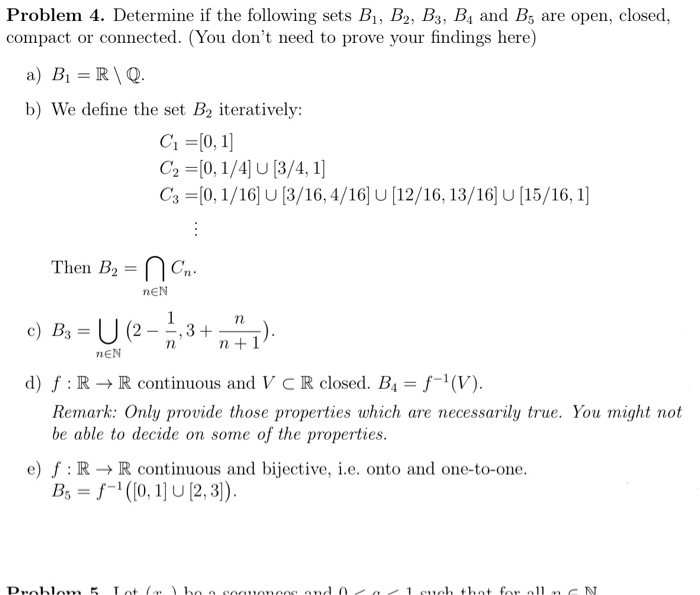Problem 4. Determine if the following sets B1, B2, B3, B4 and Bs are open, closed, compact or connected. (You don't need to prove your findings here) a) B1 =RQ. b) We define the set B2 iteratively: C1 = [0, 1] C2 =[0,1/4] U [3/4, 1] C3 =[0,1/16] U [3/16, 4/16] U [12/16, 13/16] U [15/16, 1] Then B2 = n Cn. NEN c) B3 = U (2-7,3+"). nn +1 NEN d) f:R+R continuous and V CR closed. B4 =...

• ### Find the matrix of the linear transformation T: V →W relative to B and C. Suppose...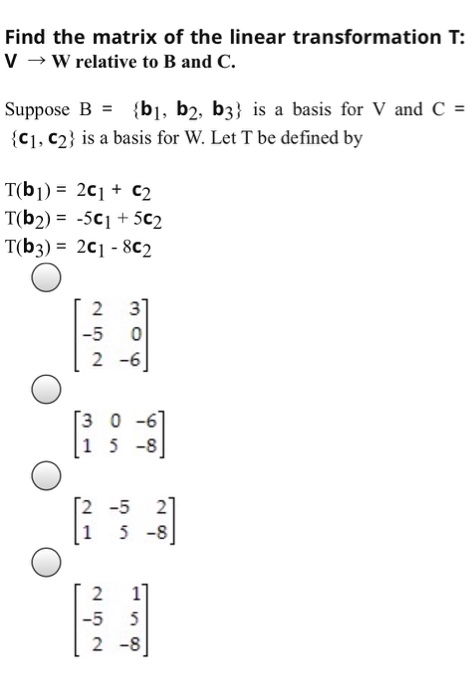Find the matrix of the linear transformation T: V →W relative to B and C. Suppose B = {bı, b2, b3} is a basis for V and C = {C1, C2} is a basis for W. Let T be defined by T(b]) = 261 + C2 T(62) = -501 +502 T(b3) = 2C1-802 2. 3 0 2 -6 [3 0 -6 1 5-8 2 -5 2 5 -8 2 1 -5 5 2 -8

• ### Let B = {b1,b2} and C= {(1,62} be bases for R2. Find the change-of-coordinates matrix from...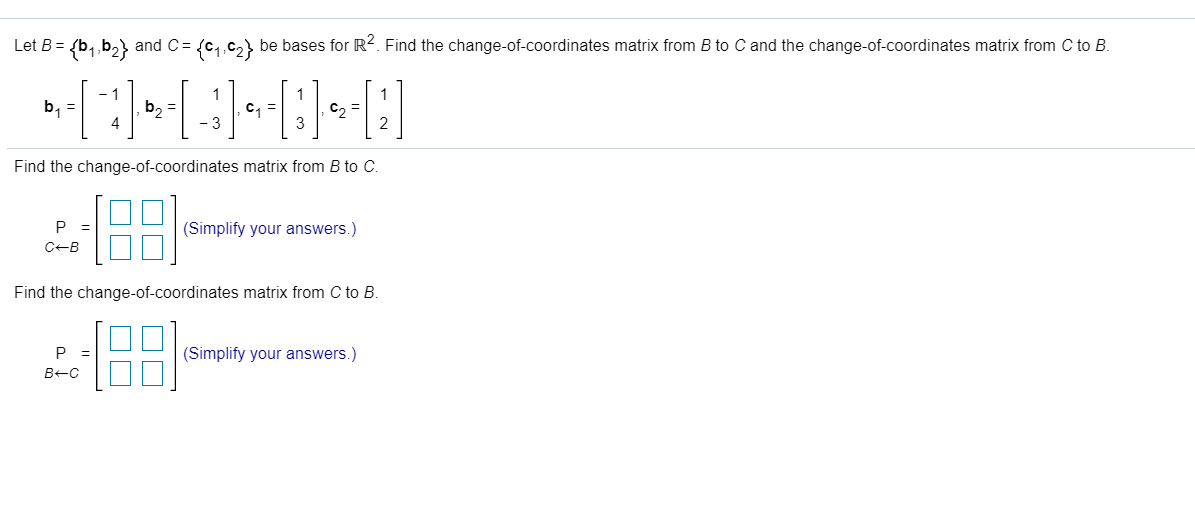Let B = {b1,b2} and C= {(1,62} be bases for R2. Find the change-of-coordinates matrix from B to C and the change-of-coordinates matrix from C to B. - 1 b = b2 = C1 = C = 4 -3 Find the change-of-coordinates matrix from B to C. P = CB (Simplify your answers.) Find the change-of-coordinates matrix from C to B. P B-C [8: (Simplify your answers.)

• ### 1. Let B1 = {1, æ, } and B2 = {x - 1,22 - 1 -...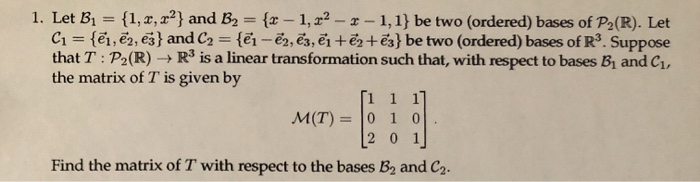1. Let B1 = {1, æ, } and B2 = {x - 1,22 - 1 - 1,1} be two (ordered) bases of P2(R). Let C1 = {ēm, ēm, ēj} and C2 = {@i - ēm, ēz, ?i + ?2+ēz} be two (ordered) bases of R3. Suppose the matrix of T is given by [1 1 1] [2 0 1 Find the matrix of T with respect to the bases B, and C.